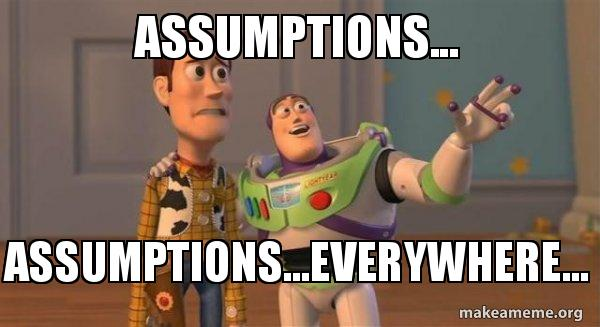## What are the key assumptions of linear regression models?

7 Jun, 2021 at 22:08 | Posted in Statistics & Econometrics | 3 Comments

In Andrew Gelman’s and Jennifer Hill’s Data Analysis Using Regression and Multilevel/Hierarchical Models the authors list the assumptions of the linear regression model. The assumptions — in decreasing order of importance — are:1. Validity. Most importantly, the data you are analyzing should map to the research question you are trying to answer. This sounds obvious but is often overlooked or ignored because it can be inconvenient. . . .

2. Additivity and linearity. The most important mathematical assumption of the regression model is that its deterministic component is a linear function of the separate predictors . . .

3. Independence of errors. . . .

4. Equal variance of errors. . . .

5. Normality of errors. . . .

Further assumptions are necessary if a regression coefficient is to be given a causal interpretation …

Yours truly can’t but concur — especially on the “decreasing order of importance” of the assumptions. But then, of course, one really has to wonder why econometrics textbooks almost invariably turn this order of importance upside-down and don’t have more thorough discussions on the overriding importance of Gelman/Hill’s two first points …

1.Where does it say they get to ignore the standard error? When we used to run linear regressions for market research analysis, it was just intellectual honesty to report the standard errors.

2.While I understand the source of their listing and your concurrence, and think assumption checking cannot be overemphasized, I would also emphasize that #1 is qualitatively distinct and ought to make us view 2-5 as follows: In soft sciences, a model (such as that defined by 2-5 above or some subset of them) is always composed of wrong assumptions, often if not usually wrong in ways that would make it very misleading to proceed as if the model were correct. Thus we should switch out of the traditional “assume this model” approach and instead ask and explain what the outputs mean given the reality that we don’t believe the assumptions.

One elegant answer comes from work of Huber, White and others: The fitted model is the “best” overall approximation (derivable from the data within the given model form) to what we should expect from the underlying data-generating process, where “best” is defined in terms of the data, the data generator, and the fitting method (e.g., for OLS, Euclidean distance from the model to the data; for the MLE, the Kullback-Leibler divergence). Whether the derived approximation is helpful or misleading of course depends on many context-specific aspects of the assumption failings, especially the actual loss functions of stakeholders (which of course will often vary quite a bit across stakeholders within the same problem). But the main point here is to no longer make models and their component assumptions (like 2-5) out as anything more than simplifying constraints that we impose for our convenience and can relax as needed, not as properties of nature that we treat as if facts until data make them look untenable.

•Interesting comment, Sander. Thanks. If only my fellow economists also understood that they’re in the realm of ‘soft sciences.’ Then they would perhaps not succumb quite as often to the overconfidence trap that you so succinctly described in your 2017 EJE article: “Overconfident inferences follow when the hypothetical nature of these inputs is forgotten and the resulting outputs are touted as unconditionally sound scientific inferences instead of the tentative suggestions that they are (however well informed) …” 🙂

Sorry, the comment form is closed at this time.